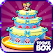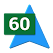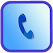Reviews Timeline
LanguagesDescription
Free app for the conversion of currencies, metric and imperial units of measurement. The following types of measure units can be converted... Area: acre, are, hectare (ha), square centimeter (cm2), square decimeter (dm2), square foot (ft2), square kilometer (km2), square meter (m2), square mile (mi2), square millimeter (mm2) and square yard (yd2).Energy: British Thermal Unit (BTU), calorie (IST), calorie (thermo), cubic centimeter-atmosphere (cc-atm), foot-pound-force (lbf), gram-calorie, inch-pound-force, Joule, kilocalorie (kcal), kilogram-calorie, kilowatt per hour (kWh), pound-CHU, US horsepower per hour and watt per hour.Flowrate: barrel per day, cubic centimeter per second (cc/s), cubic foot per minute, cubic foot per...
Mentionsdeals.woot.com##### 1.2 metric to imperial conversions - SlideShare
slideshare.net
Top ReviewedRecently Reviewed